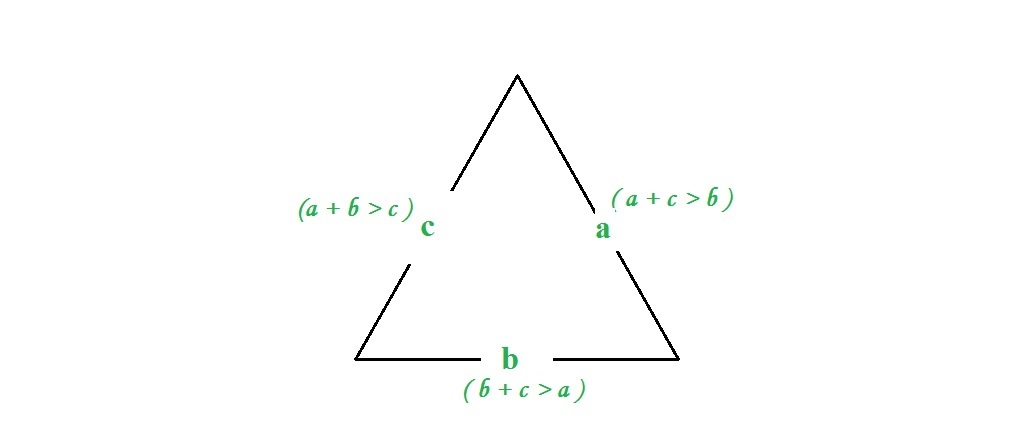# Python | Checking triangular inequality on list of lists

• Last Updated : 03 Mar, 2019

Given a list of lists, the task is to find whether a sublist satisfies the triangle inequality.

The triangle inequality states that for any triangle, the sum of the lengths of any two sides must be greater than or equal to the length of the remaining side. In other words, a triangle is valid if sum of its two sides is greater than the third side. If three sides are a, b and c, then three conditions should be met.

Attention geek! Strengthen your foundations with the Python Programming Foundation Course and learn the basics.

To begin with, your interview preparations Enhance your Data Structures concepts with the Python DS Course. And to begin with your Machine Learning Journey, join the Machine Learning - Basic Level Course

```a + b > c
a + c > b
b + c > a
```Method #1 : Using List comprehension

 `# Python code to find whether a sublist ``# satisfies the triangle inequality.`` ` `# List initialization``Input` `=` `[[``1``, ``3``, ``1``], [``4``, ``5``, ``6``]]`` ` `# Sorting sublist``for` `elem ``in` `Input``:``    ``elem.sort()`` ` `# Using list comprehension``Output ``=` `[(p, q, r) ``for` `p, q, r ``in` `Input` `if` `(p ``+` `q)>``=` `r]`` ` `# Printing output``print``(Output)`
Output:
```[(4, 5, 6)]
```

Method #2 : Using Iteration

 `# Python code to find whether a sublist``# satisfies the triangle inequality.`` ` `# List initialization``Input` `=` `[[``1``, ``1``, ``3``], [``4``, ``5``, ``6``]]`` ` `# Sorting sublist of list of list``for` `elem ``in` `Input``:``    ``elem.sort()`` ` `# Checking for triangular inequality``for` `elem ``in` `Input``:``    ``if` `elem[``0``] ``+` `elem[``1``] > elem[``2``]:``        ``print``(elem)`
Output:
```[4, 5, 6]
```

My Personal Notes arrow_drop_up